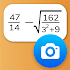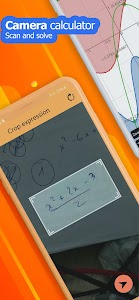# Camera math calculator - Take photo to solve v5.3.6.72

Downloads: 1184

Free

### Camera math calculator - Take photo to solve / Screenshots### Camera math calculator - Take photo to solve / Description

Math calculator solves maths problem by taking photo!

Math calculator is a useful and advanced calculator pro with basic calculator free equation solver and scientific calculator. This stylish calculator is also an equation solver and a great maths homework solver which can solve maths problems more efficiently by CAMERA .

Let's see what's inside:
➡️ Maths Camera: Good maths homework helper which solves maths problems by taking photo.
➡️ Advanced scientific Calculator: Multifunctional and scientific.
➡️ Equation calculator: Solve your maths homework efficiently.

👍 Math calculator camera - photo calculator
We provide a mathematics solutions in a creative way. While you are doing maths home work try our calculator so that you can solve maths problems more easily by taking photo and get homework answers quickly. It's a homework solver. Both basic maths problem and equations are suitable.

👍 Scientific Calculator Plus
Scientific calculator is an advanced calculator for student and engineering. Advanced calculator pro can calculate everything from basic math such as pre-algebra to advanced calculation calculus. It's an easy-to-use calculator. This scientific calculator contains all scientific calculations such as trigonometric power logarithmic square root cube root factorial π calculation.

👍 Equation calculator
There is a equation solver designed for students who need to solve equations and inequalities. What’s more you can do decimal calculation and power calculation in the equation calculator.

Show More >

### Camera math calculator - Take photo to solve / What's New in v5.3.6.72

Improved math scanner.
Added matrix menu to main screen. (Shift 4)
Instant result for equation.